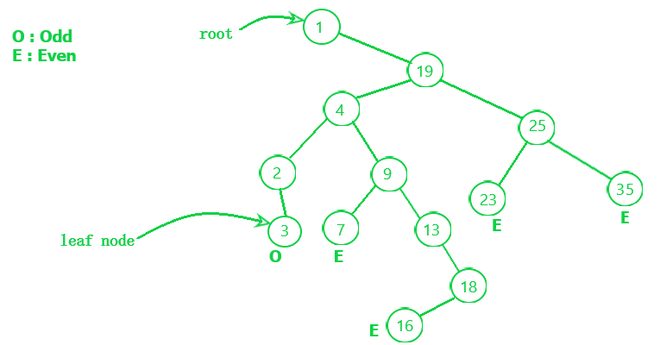# Find all even sum paths in given Binary Search Tree

• Difficulty Level : Medium
• Last Updated : 23 May, 2022

Given a Binary search tree having N nodes, the task is to find all the paths starting at the root and ending at any leaf and having an even sum.

Examples:

Input:Img-Btree

Output:
Even sum Paths are:
1st) 1 -> 19 -> 4 -> 9 -> 7 = sum(40)
2nd) 1 -> 19 -> 4 -> 9 -> 13 -> 18 -> 16 = sum(80)
3rd) 1 -> 19 -> 25 -> 35 = sum(68)
4th) 1 -> 19 -> 25 -> 23 = sum(80)
Explanation: When we start traversing form root node to the leaf node then we have different path are (1, 19, 4, 9, 7), (1, 19, 4, 9, 13, 18, 16), (1, 19, 25, 35), (1, 19, 25, 23) and (1, 19, 4, 2, 3). And after finding the sum of every path we found that all are even. Here we found one path as odd which are 1 -> 19 -> 4 -> 2 -> 3 = sum(29).

Input:    2
/  \
1     3
Output: No path
Explanation: There is no path which has even sum.

Approach: The problem can be solved using a stack, based on the following idea:

Traverse the whole tree and keep storing the path in the stack. When the leaf is reached check if the sum of the path is even or not.

Follow the steps mentioned below to implement the approach:

• Take one stack (say path) to store the current path.
• Recursively traverse the tree and in each iteration:
• Store the sum of all the nodes along this path (say sum) and store that node data in path also.
• Traverse for the left child.
• Traverse for the right child.
• If a leaf node is reached, check if the sum of the path is even or odd.
• Return all such paths.

Below is the code to understand better:

## C++

 `// C++ code to implement the approach` `#include ` `using` `namespace` `std;` `int` `cnt;` `// Structure of a tree node``class` `Node {``public``:``    ``Node* left;``    ``int` `val;``    ``Node* right;` `    ``Node(``int` `val)``    ``{``        ``this``->right = right;``        ``this``->val = val;``        ``this``->left = left;``    ``}``};` `// Function to print path``void` `print(stack<``int``> pth)``{``    ``vector<``int``> v;``    ``cout << ``"["``;``    ``while` `(!pth.empty()) {``        ``v.push_back(pth.top());``        ``pth.pop();``    ``}``    ``while` `(v.size() != 1) {``        ``cout << v.back() << ``","``;``        ``v.pop_back();``    ``}``    ``cout << v.back();``    ``cout << ``"]"``;``}` `// Function to find the paths``void` `cntEvenPath(Node* root, ``int` `sum, stack<``int``>& path)``{``  ` `    ``// Add the value of node in sum``    ``sum += root->val;` `    ``// Keep storing the node element``    ``// in the path``    ``path.push(root->val);` `    ``// If node has no left and right child``    ``// and also the sum is even``    ``// then return the path``    ``if` `((sum & 1) == 0``        ``&& (root->left == NULL && root->right == NULL)) {``        ``cnt++;``        ``cout << ``"Sum Of "``;``        ``print(path);``        ``cout << ``" = "` `<< sum << ``"\n"``;``        ``return``;``    ``}` `    ``// Check left child``    ``if` `(root->left != NULL) {``        ``cntEvenPath(root->left, sum, path);``        ``path.pop();``    ``}` `    ``// Check right child``    ``if` `(root->right != NULL) {``        ``cntEvenPath(root->right, sum, path);``        ``path.pop();``    ``}``}` `// Driver code``int` `main()``{``    ``// Build a tree``    ``Node* root = ``new` `Node(1);``    ``root->right = ``new` `Node(19);``    ``root->right->right = ``new` `Node(25);``    ``root->right->left = ``new` `Node(4);``    ``root->right->left->left = ``new` `Node(2);``    ``root->right->left->right = ``new` `Node(9);``    ``root->right->right->left = ``new` `Node(23);``    ``root->right->right->right = ``new` `Node(35);``    ``root->right->left->left->right = ``new` `Node(3);``    ``root->right->left->right->left = ``new` `Node(7);``    ``root->right->left->right->right = ``new` `Node(13);``    ``root->right->left->right->right->right = ``new` `Node(18);``    ``root->right->left->right->right->right->left``        ``= ``new` `Node(16);` `    ``cout << ``"\n"``;` `    ``// Stack to store path``    ``stack<``int``> path;``    ``cntEvenPath(root, 0, path);``    ``if` `(cnt == 0)``        ``cout << ``"No path"``;``    ``return` `0;``}` `// This code is contributed by Rohit Pradhan`

## Java

 `// Java code to implement the approach` `import` `java.io.*;``import` `java.lang.*;``import` `java.util.*;` `class` `GFG {``    ``static` `int` `count = ``0``;` `    ``// Structure of a tree node``    ``static` `class` `Node {``        ``Node left;``        ``int` `val;``        ``Node right;` `        ``Node(``int` `val)``        ``{``            ``this``.right = right;``            ``this``.val = val;``            ``this``.left = left;``        ``}``    ``}` `    ``// Function to find the paths``    ``static` `void` `cntEvenPath(Node root, ``int` `sum,``                            ``Stack path)``    ``{``        ``// Add the value of node in sum``        ``sum += root.val;` `        ``// Keep storing the node element``        ``// in the path``        ``path.push(root.val);` `        ``// If node has no left and right child``        ``// and also the sum is even``        ``// then return the path``        ``if` `((sum & ``1``) == ``0``            ``&& (root.left == ``null``                ``&& root.right == ``null``)) {``            ``count++;``            ``System.out.println(``"Sum Of"``                               ``+ path + ``" = "``                               ``+ sum);``            ``return``;``        ``}` `        ``// Check left child``        ``if` `(root.left != ``null``) {``            ``cntEvenPath(root.left, sum, path);``            ``path.pop();``        ``}` `        ``// Check right child``        ``if` `(root.right != ``null``) {``            ``cntEvenPath(root.right, sum, path);``            ``path.pop();``        ``}``    ``}` `    ``// Driver code``    ``public` `static` `void` `main(String[] args)``    ``{``        ``// Build a tree``        ``Node root = ``new` `Node(``1``);``        ``root.right = ``new` `Node(``19``);``        ``root.right.right = ``new` `Node(``25``);``        ``root.right.left = ``new` `Node(``4``);``        ``root.right.left.left = ``new` `Node(``2``);``        ``root.right.left.right = ``new` `Node(``9``);``        ``root.right.right.left = ``new` `Node(``23``);``        ``root.right.right.right``            ``= ``new` `Node(``35``);``        ``root.right.left.left.right``            ``= ``new` `Node(``3``);``        ``root.right.left.right.left``            ``= ``new` `Node(``7``);``        ``root.right.left.right.right``            ``= ``new` `Node(``13``);``        ``root.right.left.right.right.right``            ``= ``new` `Node(``18``);``        ``root.right.left.right.right.right.left``            ``= ``new` `Node(``16``);` `        ``System.out.println();` `        ``// Stack to store path``        ``Stack path = ``new` `Stack<>();``        ``cntEvenPath(root, ``0``, path);``        ``if` `(count == ``0``)``            ``System.out.println(``"No path"``);``    ``}``}`

## Python3

 `# Python code to implement the approach` `# Node to create the tree``class` `Node:``    ``def` `__init__(``self``, val):``        ``self``.right ``=` `None``        ``self``.val ``=` `val``        ``self``.left ``=` `None` `# Function to find the paths``def` `cntEvenPath(root, ``sum``, path):``  ` `    ``# Add the value of node in sum``    ``sum` `+``=` `root.val` `    ``# Keep storing the node element``    ``# in the path``    ``path.append(root.val)` `    ``# If node has no left and right child``    ``# and also the sum is even``    ``# then return the path``    ``if` `((``sum` `& ``1``) ``=``=` `0` `and` `root.left ``=``=` `None` `and` `root.right ``=``=` `None``):``        ``global` `count``        ``count ``+``=` `1``        ``print``(``"Sum Of"``, path, ``" = "``, ``sum``)``        ``return` `    ``# Check left child``    ``if` `(root.left !``=` `None``):``        ``cntEvenPath(root.left, ``sum``, path)``        ``path.pop()` `    ``# Check right child``    ``if` `(root.right !``=` `None``):``        ``cntEvenPath(root.right, ``sum``, path)``        ``path.pop()` `count ``=` `0` `# Defining main function``def` `main():``    ``# Build a tree``    ``root ``=` `Node(``1``)``    ``root.right ``=` `Node(``19``)``    ``root.right.right ``=` `Node(``25``)``    ``root.right.left ``=` `Node(``4``)``    ``root.right.left.left ``=` `Node(``2``)``    ``root.right.left.right ``=` `Node(``9``)``    ``root.right.right.left ``=` `Node(``23``)``    ``root.right.right.right ``=` `Node(``35``)``    ``root.right.left.left.right ``=` `Node(``3``)``    ``root.right.left.right.left ``=` `Node(``7``)``    ``root.right.left.right.right ``=` `Node(``13``)``    ``root.right.left.right.right.right ``=` `Node(``18``)``    ``root.right.left.right.right.right.left ``=` `Node(``16``)` `    ``# Stack to store path``    ``path ``=` `[]``    ``cntEvenPath(root, ``0``, path)``    ``if` `(count ``=``=` `0``):``        ``print``(``"No path"``)`  `if` `__name__ ``=``=` `"__main__"``:``    ``main()` `# This code is contributed by jainlovely450`

Output

```Sum Of [1,19,4,9,7] = 40
Sum Of [1,19,4,9,13,18,16] = 80
Sum Of [1,19,25,23] = 68
Sum Of [1,19,25,35] = 80```

Time Complexity: O(N)
Auxiliary Space: O(N)

My Personal Notes arrow_drop_up